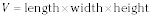##### Geometry: 1,001 Practice Problems For Dummies (+ Free Online Practice)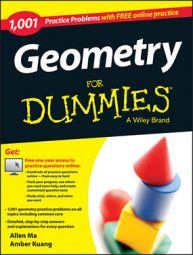Many formulas are associated with the study of three-dimensional shapes in geometry. Here, you find formulas for calculating the volume, surface area, and lateral area of cylinders, cones, spheres, pyramids, cube, and rectangular prisms.

## Cylinders

• The lateral area of a cylinder equals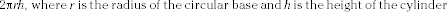•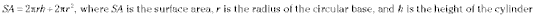•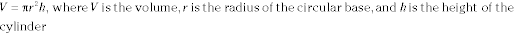## Cones

• The lateral area equals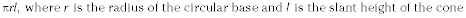•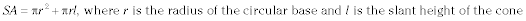•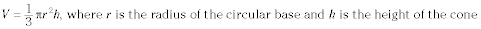## Spheres

•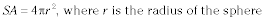•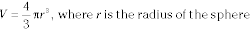## Square pyramids

•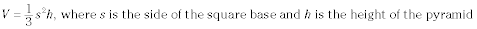## Cubes

•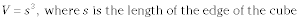## Rectangular prisms

•